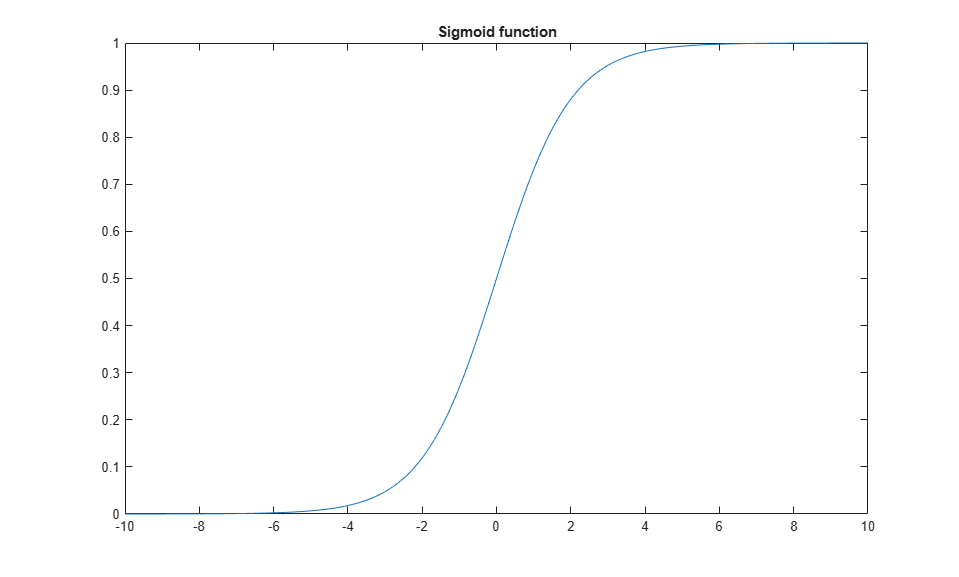# Generate HDL-Compatible Lookup Table Function Replacements Using coder.approximate

This example shows MATLAB® code generation from a floating-point MATLAB design that is not ready for code generation. Use `coder.approximate` function to generate a lookup table based MATLAB function. This newly generated function is ready for HDL code generation (not shown in this demo).

### Introduction

The MATLAB code used in the example is sigmoid function, which is used for threshold detection and decision making problems. For example, neural networks use sigmoid functions with appropriate thresholds to train systems for learning patterns.

### MATLAB Design

```design_name = 'mlhdlc_approximate_sigmoid'; testbench_name = 'mlhdlc_approximate_sigmoid_tb'; ```

This function call displays the file contents of `design_name`.

```dbtype(design_name) ```
```1 function y = mlhdlc_approximate_sigmoid( x ) 2 % 3 4 % Copyright 2014-2015 The MathWorks, Inc. 5 6 y = 1./(1+exp(-x)); 7 end ```

### Simulate the Design

It is always a good practice to simulate the design with the testbench prior to code generation to make sure there are no runtime errors.

```mlhdlc_approximate_sigmoid_tb ```Use `coder.approximate` to generate a lookup-table based replacement function for `mlhdlc_approximate_sigmoid`.

### Generate fixed-point lookup-table replacements

```repCfg = coder.approximation('Function','mlhdlc_approximate_sigmoid','CandidateFunction',@mlhdlc_approximate_sigmoid,... 'NumberOfPoints',50,'InputRange',[-10,10],'FunctionNamePrefix','repsig_'); coder.approximate(repCfg); ```

First the fixed-point conversion completes with appropriate function replacements, and following console message,

```### Generating approximation for 'sigmoid' : repsiglookuptable.m ### Generating testbench for 'sigmoid' : repsiglookuptable_tb.m ### LookupTable replacement for function 'sigmoid' used 50 data points```

This should generate the MATLAB files `repsig_lookuptable_tb`, and `repsig_lookuptable` containing the testbench and design respectively.

### Test the replacement functions

To visually see the degree of match between lookup-table based replacement function and the original function use the testbench,

```repsig_lookuptable_tb(); ```